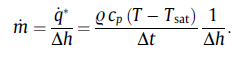## Fluids

•Navsing
Subscriber
Hi,nnI am conducting a 2D simulation of water-vapor condensation upon a pipe wall. My simulation runs fine when using the default Lee's model in fluent.nnHowever I'm trying to implement a mass transfer phase change model from a paper which will provide more accurate results. I'm implementing this through the UDF macro DEFINE_MASS_TRANSFER.nnAlthough when I run the simulation with my mass transfer udf, it runs for approx 100 iterations and then the simulation starts to diverge. My UDF is listed here:n#include udf.hnnDEFINE_MASS_TRANSFER(liq_gas_source, cell, thread, from_index,from_species_index, to_index, to_species_index)n{nreal m_lg;nreal T_SAT = 373.15;nThread *gas = THREAD_SUB_THREAD(thread, from_index);nThread *liq = THREAD_SUB_THREAD(thread, to_index);nreal delta_t;ndelta_t = CURRENT_TIMESTEP;nreal lc = C_H(cell,gas)-C_H(cell,liq)nnm_lg = 0.;nif (C_T(cell, liq) >= T_SAT)n{nm_lg = (1./lc)*C_VOF(cell,liq)*(C_R(cell,liq)*C_CP(cell,liq)* fabs(T_SAT-C_T(cell,liq))/delta_t);n}nelse if ((m_lg == 0. ) && (C_T(cell,gas) <= T_SAT))n{nm_lg = (1./lc)*C_VOF(cell,gas)*(C_R(cell,gas)*C_CP(cell,gas)* fabs(T_SAT-C_T(cell,gas))/delta_t);n}nnreturn (m_lg);n}nnAny ideas of what could be causing this?.Many thanks!n
•YasserSelima
Subscriber
Hello,nI am not sure if this is right or not, but I just noticed that m_lg is positive in both cases .. I believe that one case should be negative.nYassern
•Navsing
Subscriber
Hello YassernYeah I didn't set this as I will only have condensation. But I did try to set m_lg for condensation negative and it still crashed at roughly the same amount of iterations.
•YasserSelima
Subscriber
I believe this is expected because of using high coefficient 1 ... Not sure how you can solve the problem but you can try decreasing the timestep, decreasing the under-relaxtaion factors and increasing the number of iterations in every timestepn
•Navsing
Subscriber
I have tried to decrease the timestep, relaxation factors and increase the iterations per timestep, still have the same problem.nWhat do you mean by using the high coeffficeint 1? Should I re-write the equation?n
•YasserSelima
Subscriber
I mean this 1nm_lg = ( 1. /lc)*C_VOF(cell,liq)*(C_R(cell,liq)*C_CP(cell,liq)* fabs(T_SAT-C_T(cell,liq))/delta_t);nnthe solver will keep transferring almost all the liquid to vapour in one iteration, then all the vapour to liquid in the next .... the solution is something in between n
•Navsing
Subscriber
Thats just 1/Latent heat of vaporisation. I'm using this formula to determine the mass transfer:nnn
•YasserSelima
Subscriber
yes, I do understand the equation ... but what happens is when you have all the liquid in the cell evaporating, the temperature decreases ... this causes the solver to re-apply the equation and condenses all the vapour ... the temperature in the cell increases ... and so on. In addition to having energy balance between the cells, this becomes a a tough process for the solver to reach solution.nWhat happens in reality is ... the temperature increases, then part of the liquid evaporates until the temperature reaches saturation ... not all the liquid. So, the coefficient should not be 1 ... The default value is 0.1 .. you can increase it to 0.2 .. 0.5 ... but 1 will end up with numerical instability n